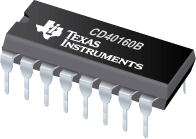# Logic Counters InformationLogic counters are integrated circuits (ICs) that count events in computers and other digital systems. Because they must remember past states, logic counters include memory. Generally, logic counters consist of bistable devices or bistable multivibrators called flip-flops. The number of flip-flops and the way in which they are connected determines the number of states and the sequence of states that logic counters complete in each full cycle.

## Types of Logic Counters

The Engineering360 SpecSearch Database contains information and searchable data on the various functional types of logic counters.

• Binary coded decimal (BCD) counters use a four-bit code to represent each digit of a decimal number.
• Decade counters are four-bit devices that produce a counting sequence from 00002 (0) to 10012 (9).
• MOD-N counters come in truncated and non-truncated versions. For truncated counters, the MOD (modulus) number is given as 2n, where n is the number of stages of the counter. Truncated counters (also known as divide-by-N counters) n represents the total number of different counting states.
• Bi-quinary counters are decade counters in which the most significant bit (MSB) is low for five states and high for the other five, producing a 50% duty cycle in the form of a square wave output.

## Specifications

A number of other specifications for logic counters should be considered other than or in addition to the desired device type.

### Synchronous vs. Asynchronous

When selecting logic counters, it is important to specify whether the device is synchronous or asynchronous. Synchronous / asynchronous counters can operate as either type.

Synchronous counters provide output bits that change state simultaneously, with no ripple. These devices must be designed using connected J-K flip-flops, where each flip-flop receives the exact same clock pulse at the exact same time.

In asynchronous counters, the external clock pulse clocks the first flip-flop, and then each successive flip-flop is clocked by one of the outputs of the previous flip-flop.

### Counting Direction

Digital counters can count either up, down, or both up and down in sequence. Up counters advance the count forward, such as from 1 to 2. Down counters move the count backward, such as from 2 to 1. Bi-directional counters can count forward or backward in sequence, depending on an application’s requirements.

### Logic

Selecting logic counters requires an analysis of logic families. Transistor-transistor logic (TTL) and related technologies such as Fairchild advanced Schottky TTL (FAST) use transistors as digital switches. By contrast, emitter coupled logic (ECL) uses transistors to steer current through gates that compute logical functions. Another logic family, complementary metal-oxide semiconductor (CMOS), uses a combination of p-type and n-type metal-oxide-semiconductor field effect transistors (MOSFETs) to implement logic gates and other digital circuits. Logic families for counters include cross-bar switch technology (CBT), Gallium arsenide (GaAs), integrated injection logic (I2L) and silicon on sapphire (SOS). Gunning with transceiver logic (GTL) and gunning with transceiver logic plus (GTLP) are also available.

#### Image credit:

Texas Instruments